# Excel FILTER function with formula examples

In this quick lesson, you will learn how to filter in Excel dynamically with formulas. Examples to filter duplicates, cells containing certain text, with multiple criteria, and more.

How do you usually filter in Excel? For the most part, by using Auto Filter, and in more complex scenarios with Advanced Filter. Being fast and powerful, these methods have one significant drawback - they do not update automatically when your data changes, meaning you would have to clean up and filter again. The introduction of the FILTER function in Excel 365 becomes a long-awaited alternative to the conventional features. Unlike them, Excel formulas recalculate automatically with each worksheet change, so you'll need to set up your filter just once!

## Excel FILTER function

The FILTER function in Excel is used to filter a range of data based on the criteria that you specify.

The function belongs to the category of Dynamic Arrays functions. The result is an array of values that automatically spills into a range of cells, starting from the cell where you enter a formula.

The syntax of the FILTER function is as follows:

FILTER(array, include, [if_empty])

Where:

• Array (required) - the range or array of values that you want to filter.
• Include (required) - the criteria supplied as a Boolean array (TRUE and FALSE values).
Its height (when data is in columns) or width (when data is in rows) must be equal to the that of the array argument.
• If_empty (optional) - the value to return when no entries meet the criteria.

The FILTER function is only available in Excel for Microsoft 365 and Excel 2021. In Excel 2019, Excel 2016 and earlier versions, it is not supported.

## Basic Excel FILTER formula

For starters, let's discuss a couple of very simple cases just to gain more understanding how an Excel formula to filter data works.

From the below data set, supposing you want to extract the records with a specific value in the Group, column, say group C. To have it done, we supply the expression B2:B13="C" to the include argument, which will produce a required Boolean array, with TRUE corresponding to "C" values.

`=FILTER(A2:C13, B2:B13="C", "No results")`

In practice, it's more convenient to input the criteria in a separate cell, e.g. F1, and use a cell reference instead of hardcoding the value directly in the formula:

`=FILTER(A2:C13, B2:B13=F1, "No results")`

Unlike Excel's Filter feature, the function does not make any changes to the original data. It extracts the filtered records into the so-called spill range (E4:G7 in the screenshot below), beginning in the cell where the formula is entered: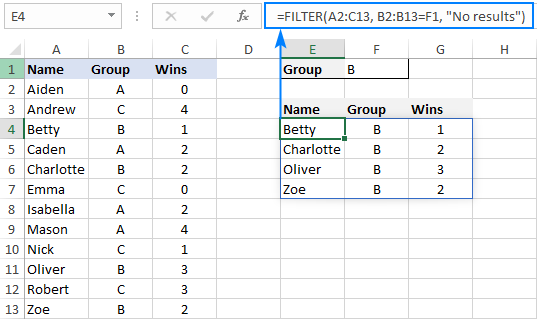If no records match the specified criteria, the formula returns the value you put in the if_empty argument, "No results" in this example: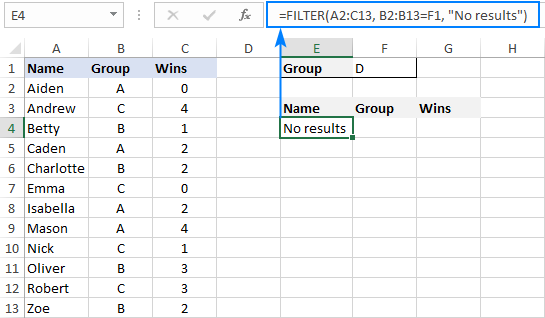If you'd rather return nothing in this case, then supply an empty string ("") for the last argument:

`=FILTER(A2:C13, B2:B13=F1, "")`

In case your data is organized horizontally from left to right like shown in the screenshot below, the FILTER function will work nicely too. Just make sure you define appropriate ranges for the array and include arguments, so that the source array and Boolean array have the same width:

`=FILTER(B2:M4, B3:M3= B7, "No results")`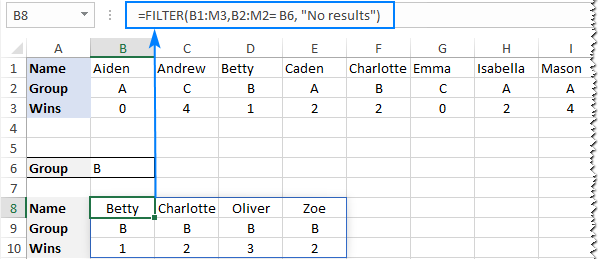### Excel FILTER function - usage notes

To effectively filter in Excel with formulas, here are a couple of important points to take notice of:

• The FILTER function automatically spills the results vertically or horizontally in the worksheet, depending on how your original data is organized. So, please make sure you always have enough empty cells down and to the right, otherwise you'll get a #SPILL error.
• The results of the Excel FILTER function are dynamic, meaning they update automatically when values in the original data set change. However, the range supplied for the array argument is not updated when new entries are added to the source data. If you wish the array to resize automatically, then convert it to an Excel table and build formulas with structured references, or create a dynamic named range.

## How to filter in Excel - formula examples

Now that you know how a basic Excel filter formula works, it's time to get some insights into how it could be extended for solving more complex tasks.

### Filter with multiple criteria (AND logic)

To filter data with multiple criteria, you supply two or more logical expressions for the include argument:

The multiplication operation processes the arrays with the AND logic, ensuring that only the records that meet all the criteria are returned. Technically, it works this way:

The result of each logical expression is an array of Boolean values, where TRUE equates to 1 and FALSE to 0. Then, the elements of all the arrays in the same positions are multiplied. Since multiplying by zero always gives zero, only the items for which all the criteria are TRUE get into the resulting array, and consequently only those items are extracted.

The below examples show this generic formula in action.

#### Example 1. Filter multiple columns in Excel

Extending our basic Excel FILTER formula a little further, let's filter the data by two columns: Group (column B) and Wins (column C).

For this, we set up the following criteria: type the name of the target group in F2 (criteria1) and the minimum required number of wins in F3 (criteria2).

Given that our source data is in A2:C13 (array), groups are in B2:B13 (range1) and wins are in C2:C13 (range2), the formula takes this form:

`=FILTER(A2:C13, (B2:B13=F2) * (C2:C13>=F3), "No results")`

As the result, you get a list of players in group A who have secured 2 or more wins: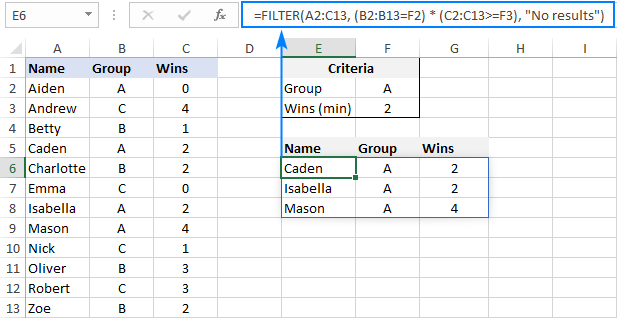#### Example 2. Filter data between dates

First off, it should be noted that it's not possible to make up a generic formula to filter by date in Excel. In different situations, you will need to build criteria differently, depending on whether you want to filter by a specific date, by month, or by year. The purpose of this example is to demonstrate the general approach.

To our sample data, we add one more column containing the dates of the last win (column D). And now, we will extract the wins that occurred in a specific period, say between May 17 and May 31.

Please notice that in this case, both criteria apply to the same range:

`=FILTER(A2:D13, (D2:D13>=G2) * (D2:D13<=G3), "No results")`

Where G2 and G3 are the dates to filter between.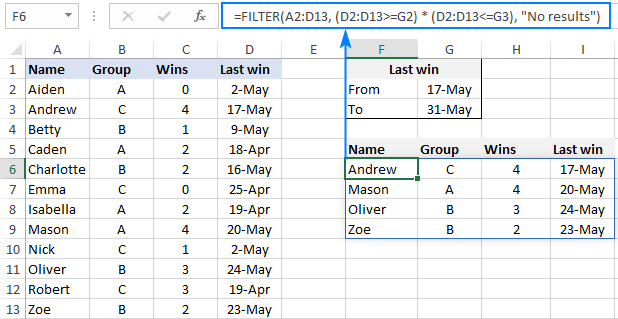### Filter with multiple criteria (OR logic)

To extract data based on multiple OR condition, you also use logical expressions like shown in the previous examples, but instead of multiplying, you add them up. When the Boolean arrays returned by the expressions are summed, the resulting array will have 0 for entries that do not meet any criteria (i.e. all the criteria are FALSE), and such entries will be filtered out. The entries for which at least one criterion is TRUE will be extracted.

Here's the generic formula to filter columns with the OR logic:

As an example, let's extract a list of players that have this or that number of wins.

Assuming the source data is in A2:C13, wins are in C2:C13, and the win numbers of interest are in F2 and F3, the formula would go as follows:

`=FILTER(A2:C13, (C2:C13=F2) + (C2:C13=F3), "No results")`

As the result, you know which players have won all the games (4) and which have won none (0):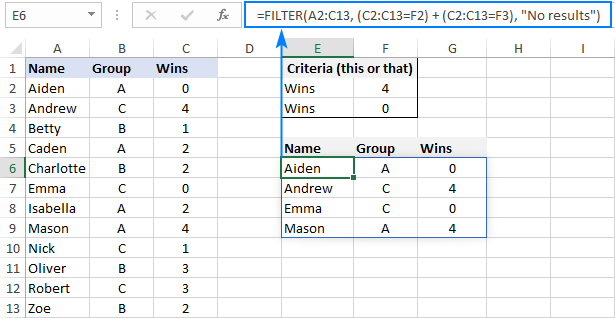### Filter based on multiple AND as well as OR criteria

In situation when you need to apply both criteria types, remember this simple rule: join the AND criteria with asterisk (*) and OR criteria with the plus sign (+).

For example, to return a list of players that have a given number of wins (F2) AND belong to the group mentioned in either E2 OR E3, build the following chain of logical expressions:

`=FILTER(A2:C13, (C2:C13=F2) * ((B2:B13=E2) + (B2:B13=E3)), "No results")`

And you will get the following result: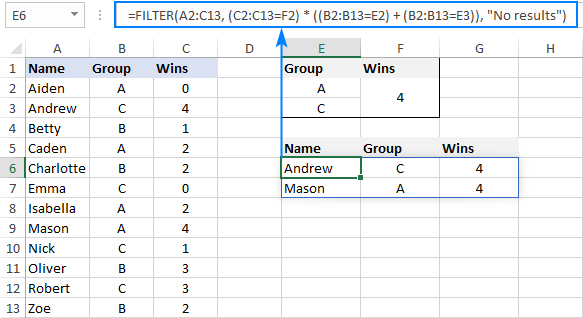### How to filter duplicates in Excel

When working with huge worksheets or combining data from different sources, there's often a possibility that some duplicates would sneak in.

If you are looking to filter out duplicates and extract unique items, then use the UNIQUE function as explained in the above linked tutorial.

If your goal is to filter duplicates, i.e. extract entries that occur more than once, then use the FILTER function together with COUNTIFS.

The idea is to get the occurrences counts for all the records and extract those greater than 1. To get the counts, you supply the same range for each criteria_range / criteria pair of COUNTIFS like this:

For example, to filter duplicate rows from the data in A2:C20 based on the values in all 3 columns, here's the formula to use:

`=FILTER(A2:C20, COUNTIFS(A2:A20, A2:A20, B2:B20, B2:B20, C2:C20, C2:C20)>1, "No results")`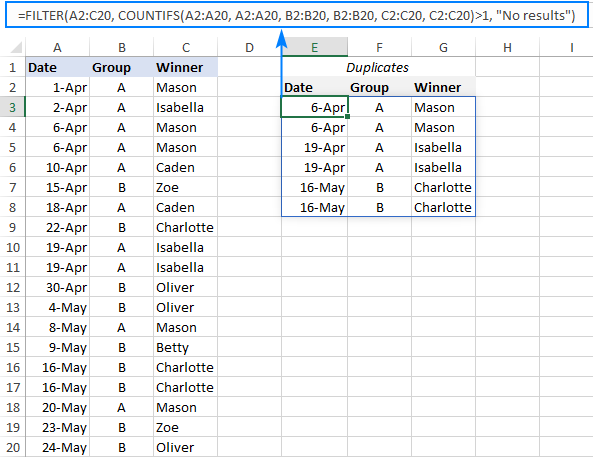Tip. To filter duplicates based on the values in the key columns, include only those specific columns in the COUNTIFS function.

### How to filter out blanks in Excel

A formula for filtering out blank cells is, in fact, a variation of the Excel FILTER formula with multiple AND criteria. In this case, we check whether all (or particular) columns have any data in them and exclude the rows where at least one cell is empty. To identify non-blank cells, you use the "not equal to" operator (<>) together with an empty string ("") like this:

With the source data in A2:C12, to filter out rows containing one or more blank cells, the following formula is entered in E3: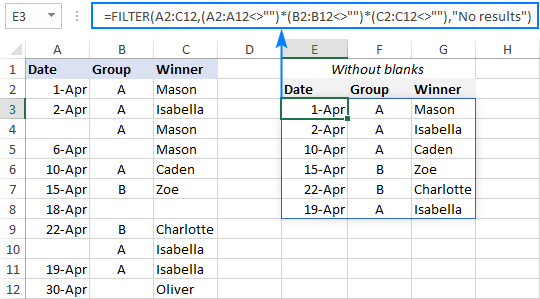### Filter cells containing specific text

To extract cells that contain certain text, you can use the FILTER function together with the classic If cell contains formula:

Here's how it works:

• The SEARCH function looks for a specified text string in a given range and returns either a number (the position of the first character) or #VALUE! error (text not found).
• The ISNUMBER function converts all the numbers to TRUE and errors to FALSE and passes the resulting Boolean array to the include argument of the FILTER function.

For this example, we've added the Last names of players in B2:B13, typed the part of the name we want to find in G2, and then use the following formula to filter the data:

`=FILTER(A2:D13, ISNUMBER(SEARCH(G2, B2:B13)), "No results")`

As the result, the formula retrieves the two surnames containing "han":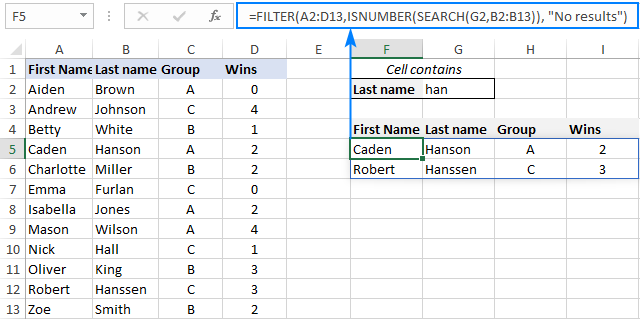### Filter and calculate (Sum, Average, Min, Max, etc.)

A cool thing about the Excel FILTER function is that it can not only extract values with conditions, but also summarize the filtered data. For this, combine FILTER with aggregation functions such as SUM, AVERAGE, COUNT, MAX or MIN.

For instance, to aggregate data for a specific group in F1, use the following formulas:

Total wins:

`=SUM(FILTER(C2:C13, B2:B13=F1, 0))`

Average wins:

`=AVERAGE(FILTER(C2:C13, B2:B13=F1, 0))`

Maximum wins:

`=MAX(FILTER(C2:C13, B2:B13=F1, 0))`

Minimum wins:

`=MIN(FILTER(C2:C13, B2:B13=F1, 0))`

Please pay attention that, in all the formulas, we use zero for the if_empty argument, so the formulas would return 0 if no values meeting the criteria are found. Supplying any text such as “No results” would result in a #VALUE error, which is obviously the last thing you want :)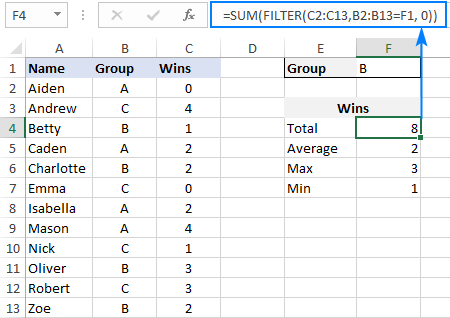### Case-sensitive FILTER formula

A standard Excel FILTER formula is case-insensitive, meaning it makes no distinction between lowercase and uppercase characters. To distinguish text case, nest the EXACT function in the include argument. This will force FILTER to do logical test in a case-sensitive manner:

Supposing, you have both groups A and a and wish to extract records where the group is the lowercase "a". To have it done, use the following formula, where A2:C13 is the source data and B2:B13 are groups to filter:

`=FILTER(A2:C13, EXACT(B2:B13, "a"), "No results")`

As usual, you can input the target group in a predefined cell, say F1, and use that cell reference instead of hardcoded text:

`=FILTER(A2:C13, EXACT(B2:B13, F1), "No results")`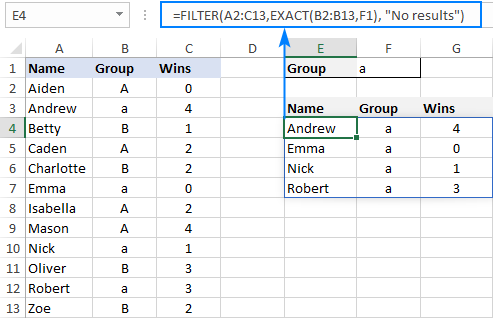## How to FILTER data and return only specific columns

For the most part, filtering all columns with a single formula is what Excel users want. But if your source table contains tens or even hundreds of columns, you may certainly want to limit the results to a few most important ones.

### Example 1. Filter some adjacent columns

In situation when you want some neighboring columns to appear in a FILTER result, include only those columns in array because it is this argument that determines which columns to return.

In the basic FILTER formula example, supposing you wish to return the first 2 columns (Name and Group). So, you supply A2:B13 for the array argument:

`=FILTER(A2:B13, B2:B13=F1, "No results")`

As the result, we get a list of participants of the target group defined in F1: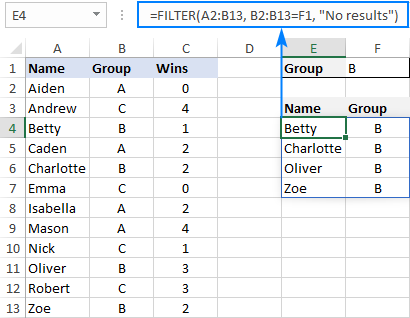### Example 2. Filter non-adjacent columns

To cause the FILTER function to return non-contiguous columns, use this clever trick:

1. Make a FILTER formula with the desired condition(s) using the entire table for array.
2. Nest the above formula inside another FILTER function. To configure the "wrapper" function, use an array constant of TRUE and FALSE values or 1's and 0's for the include argument, where TRUE (1) marks the columns to be kept and FALSE (0) marks the columns to be excluded.

For example, to return only Names (1st column) and Wins (3rd column), we are using {1,0,1} or {TRUE,FALSE,TRUE} for the include argument of the outer FILTER function:

`=FILTER(FILTER(A2:C13, B2:B13=F1), {1,0,1})`

Or

`=FILTER(FILTER(A2:C13, B2:B13=F1), {TRUE,FALSE,TRUE})`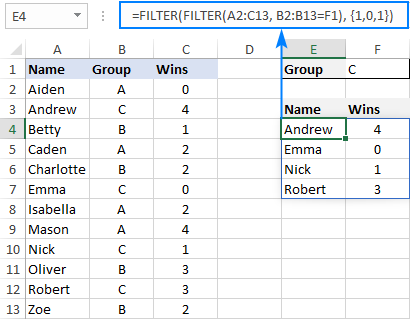## How to limit the number of rows returned by FILTER function

If your FILTER formula finds quite a lot of results, but your worksheet has limited space and you cannot delete the data below, then you can limit the number of rows the FILTER function returns.

Let's see how it works on an example of a simple formula that pulls players from the target group in F1:

`=FILTER(A2:C13, B2:B13=F1)`

The above formula outputs all the records that it finds, 4 rows in our case. But suppose you just have space for two. To output only the first 2 found rows, this is what you need to do:

• Plug the FILTER formula into the array argument of the INDEX function.
• For the row_num argument of INDEX, use a vertical array constant like {1;2}. It determines how many rows to return (2 in our case).
• For the column_num argument, use a horizontal array constant like {1,2,3}. It specifies which columns to return (the first 3 columns in this example).
• To take care of possible errors when no data matching your criteria is found, you can wrap your formula in the IFERROR function.

The complete formula takes this form:

`=IFERROR(INDEX(FILTER(A2:C13, B2:B13=F1), {1;2}, {1,2,3}), "No result")`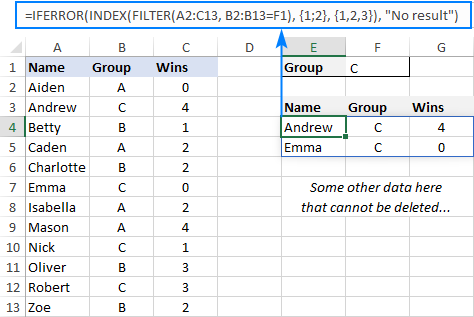When working with large tables, writing array constants manually may be quite cumbersome. No problem, the SEQUENCE function can generate the sequential numbers for you automatically:

`=IFERROR(INDEX(FILTER(A2:C13, B2:B13=F1), SEQUENCE(2), SEQUENCE(1, COLUMNS(A2:C13))), "No result")`

The first SEQUENCE generates a vertical array containing as many sequential numbers as specified in the first (and only) argument. The second SEQUENCE uses the COLUMNS function to count the number of columns in the dataset and produces an equivalent horizontal array.

Tip. To return data from specific columns, not all the columns, in the horizontal array constant that you use for the column_num argument of INDEX, include only those specific numbers. For instance, to extract data from the 1st and 3rd columns, use {1,3}.

## Excel FILTER function not working

In situation when your Excel FILTER formula results in an error, most likely that will be one of the following:

### #CALC! error

Occurs if the optional if_empty argument is omitted, and no results meeting the criteria are found. The reason is that currently Excel does not support empty arrays. To prevent such errors, be sure to always define the if_empty value in your formulas.

### #VALUE error

Occurs when the array and include argument have incompatible dimensions.

### #N/A, #VALUE, etc.

Different errors may occur if some value in the include argument is an error or cannot be converted to a Boolean value.

### #NAME error

Occurs when trying to use FILTER in an older version of Excel. Please remember that it is a new function, which is only available in Office 365 and Excel 2021.

In new Excel, a #NAME error occurs if you accidentally misspell the function's name.

### #SPILL error

Most often, this error occurs if one or more cells in the spill range are not completely blank. To fix it, just clear or delete non-empty cells. To investigate and resolve other cases, please see #SPILL! error in Excel: what it means and how to fix.

### #REF! error

Occurs when a FILTER formula is used between different workbooks, and the source workbook is closed.

That's how to filer data in Excel dynamically. I thank you for reading and hope to see you on our blog next week!

Filter in Excel with formulas (.xlsx file)

## You may also be interested in

1. I have this data where, collumn 1 is stock names, collumn 2 is activity of stock (Buy/Sell) meaning some stock were sold or bought, collumn 3; I want to create collumn 3, such that it picks the name of the stock in collumn 1, if there is no balance of stock remaining in collumn 2, how do i go about this?
Remember the stocks are bought and sold in different dates. Will appreciate any help.

• Hi! I can't see your data, but I can guess that if a stock was bought and then sold, it is written twice in column A. If you select unique values from column A, it will be a list of stock remaining. Look for the example formulas here: Excel UNIQUE function - fastest way to find unique values. If I'm not guessing, give me an example of source data and the desired result.

• Hello Alex.
Thank you very much for your reply. Here is a sample data of the table: The collumn 1; is Name of Stock. Collumn 2: Transaction, Collumn 3: Date of the Transaction. Collumn 4: Quantity.....You will notice some stocks were fully sold out. So, we only want to have a new collumn 4, which is only picking the Name of the stock with a positive value and omit out the stock that has been sold out.

STOCK: TRANSACTION: DATE: QUANTITY:
Costco Wholesale \$\$\$ : Buy: 2/5/2023: 25
T- Mobile \$\$\$: Buy: 2/19/2023: 1000
Wells Fargo \$\$\$: Buy: 2/26/2023: 40
Amazon \$\$\$: Sell: 3/19/2023: -45
Costco Wholesale \$\$\$: Sell: 4/9/2023: -25
Enphase \$\$\$: Sell: 4/16/2023: -25
T- Mobile \$\$\$: Sell: 4/23/2023: -15
Wells Fargo \$\$\$: Sell: 5/14/2023: -40

Then the expected outcome will be a collumn only showing the stocks with a balance, and it should not leave or show empty cells:

STOCK:
Amazon \$\$\$:
Enphase \$\$\$:
T- Mobile \$\$\$:

If you can share a formula that will work this out, I will be forever greatful.

Kind Regards.

• Hi! To get a table of stocks with balance, you can create a pivot table. Using the SUMIFS formula, you can calculate the balance for each individual stock. For example,

I recommend reading this guide: Excel SUMIFS and SUMIF with multiple criteria – formula examples.

• Thanks Alex.
I have tried that formula it still gives wrong answers. I mean i want the formula that will give a collumn that picks the name of the stock which has positive balance of stock, and ignore and omit out completely those stock that have zero balance.
Will appreciate.

• The formula works with the data you gave. In your Excel2019 there are no other options other than what I have written to you.

• Thanks Alex.
When i paste the formula to the collumn of the same table i shared, it gives me these values which are not correct. Kindly check it out, I might be missing out on something, kindly guide

-48
-48
-45004
-45004
-45004
-45004
-45004
0
0
0
while i am looking for an output with only NAME OF STOCK which happens to have a balance value greater than zero.

• Note that the formula is given to you for an example. I have no way of knowing what columns your data is in. Study the guide to the SUMIFS function that I recommended. If necessary, change the data references in the formula. I can help, but not do your work for you.

• Thanks Alex for the reply.
However i have gone through the page and made adjustments accordingly to map the cells and ranges correctly,but i noticed, its giving the answer as a value. But i am looking for the answer to be the NAME OF STOCK.

• Hi! In your version of Excel, you can calculate the balance for each stock in a separate column and then use Advanced Filter to select a unique list of stocks with positive balances. Read more: Excel Advanced Filter - how to create and use.
Or instead of Advanced Filter, you can use these instructions: Find unique and distinct rows.
In newer versions of Excel, this can be done with a single formula using the FILTER and UNIQUE functions as described in this guide: Filter unique values based on multiple criteria.

• Thanks Alex.
I followed the given page and formula. this is the formula i used:
=IF(SUMIFS(E:E, B:B, B2) > 0, B2, "") this was able to give me a collumn with values which are positive.
this is what result i got:

Metta \$\$

Amazon \$\$\$
Costco Wholesale \$\$\$
Enphase \$\$\$
T- Mobile \$\$\$
Wells Fargo \$\$\$

Amazon \$\$\$

Is there a formula i can use such that, it will skip these empty cells and pick the others?
Regards.

• Hello Alex.
Thank you for the guide, It finally worked fine after fine tuning the formula.
I appreciate big time.

• Hello Alex,
Again, i have this scenario: Collumn 1 has names of stock, collumn 2 has price of stock. collumn 3 has new prices of stock. I am looking a formula which looks for names in collumn 3 and matches them against names in collumn 1, if the name is identified, we pick the price indicated in collumn 2. I tried this formula, but i realised it picks some prices correctly while others it gives a wrong answer, kindly confirm if there is something i am missing from this formula:

=FILTER('Collumn 2'!B2:B125, COUNTIF(Collumn 3!B2:B125, 'Collumn 1'!A2:A125) > 0)

Regards.

• Hi! What is written in column 3 - new prices or names? I don't have your data, so I can't understand or check your formula. I can't guess which result you want.

• Hello Alex, sorry i made a slight typing error above:

i have this scenario: Collumn 1 has names of stock, collumn 2 has price of stock. collumn 3 has new names of stock. I am looking a formula which looks for names in collumn 3 and matches them against names in collumn 1, if the name is identified, we pick the price indicated in collumn 2. I tried this formula, but i realised it picks some prices correctly while others it gives a wrong answer, kindly confirm if there is something i am missing from this formula:

=FILTER('Collumn 2'!B2:B125, COUNTIF(Collumn 3!B2:B125, 'Collumn 1'!A2:A125) > 0)

• Unfortunately, without seeing your data it is difficult to give you any advice. Please provide me with an example of the source data and the expected result.

• Hello Alex.
Also its good to say that, I am using excel 2019, so the UNIQUE function and FILTER functions are not working.

Regards.

2. Can wildcards be used as filter criteria?

• Pay attention to the following paragraph of the article above: Filter cells containing specific text.

3. I have the problem that I want to use a dynamic criteria range also in filter function, but it didn't work.
formular works by its own, but I don't want to duplicate formular with copy paste, because column count changes

my idea works fine, on substraction or sumifs like:
=IFERROR(+(B3:OFFSET(B3;0;ROWS(select_prj#)-1))-(B19:OFFSET(B19;0;ROWS(select_prj#)-1));"") where select_prj# = range of elements I want to work with

also works fine:
=UNIQUE(FILTER(Einzelposten_ab_2019!S:S;(Einzelposten_ab_2019!A:A=B3)*(Einzelposten_ab_2019!AH:AH="Rev")))

but combined I get an error
I tried:
=UNIQUE(FILTER(Einzelposten_ab_2019!S:S;(Einzelposten_ab_2019!A:A=(B3:OFFSET(B3;0;ROWS(select_prj#)-1)))*(Einzelposten_ab_2019!AH:AH="Rev")))
any idea how to solve?

• Hi! I don't have your data, so I can't understand or check your formula. I can't guess which result you want. Please clarify your specific problem or provide additional information to understand what you need.

4. I want to use filter, but my 'Include' is a formula that looks at the current time of day, when it do that nothing shows up, however if i type a fixed date, rather than the formula it works?

• Hi! Unfortunately, I can't see your data and can't guess what formula you are using.

5. Hi, I want to use something like this: =FILTER(B57:M3041,ISNUMBER(SEARCH(P1:P52,J57:J3041))=FALSE) to filter a list. I want it to NOT contain any of the values in a list (P1:P52), but it only seems to be looking at the first one in the list (P1) and filtering that. How can I do this with a list of 52 things I want to filter out? Thanks!

6. Hello!

I have been using the FILTER formula for a few days now on a new spreadsheet and have the "include" argument based upon the value of a cell (a date) within the same sheet, to check for that date on a different sheet. The issue arises when I update the date in that cell, the FILTER "include" argument immediately returns me my "Not Working" error message I put in, if nothing is found. The odd part is, when I input 10/1/2023 or 10/01/2023, it returns all values of 10/1/2023 from the other sheet. When I input 10/8/2023 or 10/08/2023, I get the "Not Working" error. Nothing changes, except the value I enter in the Cell it is checking. Here is the string I am using, the cell with the date sits in T3:

=FILTER(Master!A:R,(Master!I:I=\$T\$3)+(Master!J:J=\$T\$3)+(Master!K:K=\$T\$3)+(Master!L:L=\$T\$3)+(Master!M:M=\$T\$3)+(Master!N:N=\$T\$3)+(Master!O:O=\$T\$3)+(Master!P:P=\$T\$3)+(Master!Q:Q=\$T\$3)+(Master!R:R=\$T\$3),"Not Working")

• Hi! I can't check a formula that contains unique references to your data, which I don't have. Check what format the dates in your table are written in and what format you enter the dates in T3.

• Hello, Alexander!

Yes, they are both of the same Short Date with same MM/DD/YYYY format. 10/1/2023 receives results, but 10/8/2023 and all others give me the "Not Working" which leaves me completely baffled. I cannot figure this one out.

• Hi! I can't check your formula because I don't have your data. Perhaps some of your dates are written as text. Try comparing cells that appear to be the same: =I3=T3

• Aha, it is coming up as FALSE. I will do some more digging. Thank you so much for your time and help!

7. Not sure if this is the right forum but i am trying to use an =AVERAGE formula for a column in a workbook that recalculates when that column is filtered onlycalculating using the visible columns in the dataset.

• Hi! To calculate the average based only on the filtered values, try using the AGGREGATE function. For example,

=AGGREGATE(1,5,A1:A20)

8. Hi i am trying solve a problem but stuck if anyone help it will be grateful for me.
=COUNTA(FILTER(Sheet1!\$B\$2:\$B,(Sheet1!\$N\$2:\$N>=\$L\$1)*(Sheet1!\$N\$2:\$N<=\$L\$2),(Sheet1!\$B\$2:\$B=\$C\$1)+(Sheet1!\$B\$2:\$B=\$D\$1)+(Sheet1!\$B\$2:\$B=\$E\$1)+(Sheet1!\$B\$2:\$B=\$F\$1)+(Sheet1!\$B\$2:\$B=\$G\$1),WEEKDAY(Sheet1!\$N\$2:\$N,2)=7))

here i am trying to calculate ticket created on in the social media based on date and day and this formula only for sunday i changed weekday formula based on days but the problem i faced lets date i choose sunday but rest of the ticket count show 1 but it should be 0 as i am not clicking date of monday or tuesday.

• Hi! I can't understand your formula and check it as I don't have your data. I can't guess what result you wanted to get.

9. In a specific coloum I want to filter numbers totatl of which is equal to specific number e.g, a coloum containing data like 10,20,30,40,50,60,70,80,90,100 and I want to filter cell value total of which is eqaul to 130.

10. Hello,

If have the below column with the listed rows. Each cell basically contains a string.
COL-A:
P1-P2-A-P3
P1-P2-B-P3
P1-P2-R-P3
P1-P2-Z-P3

I also have the below column and corresponding rows
COL-E:
A
B
Z

I want to use the FILTER function to filter all the rows of which the string in COL-A contains A or B or Z (= the values in the COLE-E range)
I'll need/I want to use the SEARCH function.
I want to combine the FILTER and the SEARCH function to return (=filter) the correct rows in COL-A
I don't want to hardcode anything in the FILTER function (e.g. use "or" (+) syntax as explained in this article), nor in the SEARCH function, just work with the ranges.

Could anyone describe how to get this to work, because I didn't succeeded yet.

Kind regards

11. An online Excel level 3 course, unfortunately created in 2016, refers to 'advanced filtering' and using an additional header to this.
Criteria Range being the Additional Header labels & any Criteria Ranges specified; followed by the vital blank row. Is this format now redundant in Microsoft365 Excel?

12. I am trying to filter a list of IP addresses (3700) into 3 categories. Each category contains specific IP addresses. Is there a function that will filter the full list down to only IP addresses that are included in a single category?

For example, if I have 5 IPs that represent Category A, I would like a function that filters my data to only those 5 IPs.

• Hi! See the example formula in this paragraph in the article above: Filter with multiple OR criteria.

13. Hi is there a way to filter for both horizontal and vertical criteria at the same time ? For example if i wanted to filter the data for first and A:

2021 2021 2021 2022 2022 2022
First Mid Last First Mid Last
A
B
A
A
B
B
A

• Hi! Use the FILTER function to get the required values according to the criteria in cells N1 and N2. Combine the resulting arrays using the VSTACK function and HSTACK function. Read more: Combine ranges and arrays in Excel: VSTACK & HSTACK functions. If I understand your task correctly, try the following formula:

=VSTACK(HSTACK("",FILTER(A2:G2,A2:G2=N1)), FILTER(HSTACK(A1:A14, FILTER(A1:G14,A2:G2=N1)), A1:A14=N2))

• So i actually don't need to combine two ranges together. I basically need to filter on one range but filter for the year (horizontal criteria) and then for A (vertical) criteria which seems to be causing me problems. I can filter the array for multiple horizontal criteria or multiple vertical criteria but both at the same time is returning a #VALUES error.

• I wrote the formula based on the description provided in the second comment. I don't see your actual data and can't see what the problem is. Your explanation is not very clear.

14. Hi Team, can filter function be used with Len. I am working on extracting numbers from a column that is equal to 4.Hope this makes sense.Thanks

E.g , as an example I want to filter on below and from col B only get length which is = to 4. In this Case , Project - 4444 , 1234 , 8924

Col A Col B
Project 55615
Project 4444
Project 1234
BC Code 8924

• Hi! If I understand your task correctly, try the following formula:

=FILTER(A1:B4,LEN(B1:B4)=4)

15. Hi,

I've used this formula: =INDEX(SORT(FILTER(FILTER(Sales;(Sales[Date]=30/06/2023)*(Sales[Average Days to Pay]>0));{0,1,1,0,0,0,0,0,0,0,0,0,0,0,0,0,1,1,0,0,0,0,0,0});3;-1);{1;2;3;4;5;6;7;8;9;10;11;12;13;14;15;16;17;18;19;20};{1,2,3,4})

to sort and filter a lot of data into a top 25 result sorted by column C (amount):

A B C D
3009 216232 400,217.43 EUR
3009 204712 399,277.17 EUR
3009 211030 384,253.73 EUR
3009 211030 364,468.50 EUR
3009 211030 363,289.23 EUR
3009 211030 363,289.23 EUR
3009 211030 322,569.79 EUR
3009 200227 301,204.72 EUR
3009 211030 289,376.29 EUR
3009 200227 274,188.95 EUR
3009 200227 271,246.72 EUR
3009 200227 268,498.22 EUR
3009 200227 259,159.89 EUR
3009 201135 233,208.72 EUR
3009 200227 180,504.10 EUR
3009 200227 179,657.16 EUR
3009 208294 168,588.51 EUR
3009 200227 162,729.76 EUR
3009 257345 148,199.86 EUR
3009 247673 148,164.74 EUR

Now I need that the result shows the total sum of the amounts in column C where the criteria is the customer number in column B only - not individual items. Like this:

A B C D
3009 211030 2,087,246.77 EUR
3009 200227 1,897,189.52 EUR
3009 216232 400,217.43 EUR
3009 204712 399,277.17 EUR
3009 201135 233,208.72 EUR
3009 208294 168,588.51 EUR
3009 257345 148,199.86 EUR
3009 247673 148,164.74 EUR

Any help please? How the formula should be? Thanks

• Hi! Get a list of unique values using the UNIQUE function. Formula for E1:

=UNIQUE(A1:B20)

Using the SUMIFS function, find the sum of the two criteria in each row. Formula for G1:

=SUMIFS(\$C\$1:\$C\$20,\$A\$1:\$A\$20,E1,\$B\$1:\$B\$20,F1)

16. How do I filter, based on a values in a column, which is dynamic. Example, Column headers are country names, and I need to filter data based on the country name that is entered in cell B1?

• Hi! If I understand the question correctly, you cannot select individual columns with the FILTER function. Excel filter works for values in rows. Read carefully the article below and also: Excel Filter: How to add, use and remove.

17. How to find answer when the criteria is in range.

• Hi! If I understand correctly, look at the paragraph above: Filter with multiple OR criteria

18. Referring to the above "Filter cells containing specific text" section where you filter last names for players which contain the letters "han".

If you removed the letters "han" from cell G2 and leave the cell blank (ready as a default), would all Last Names be displayed or would nothing be displayed?

• Hi! You can download the example file at the end of the article and check the result. All values will be shown.

19. I find the "Filter cells containing specific text" part very useful (using FILTER, ISNUMBER and SEARCH). I also know that XLOOKUP can be used to find a record using a partial value.

But I find that searching an array where there are multiple occurrences of the value the XLOOKUP method gives me only one result, whereas FILTER-ISNUMBER-SEARCH method gives me results showing all occurrences.

Is it possible to use XLOOKUP to return all occurrences of the value, not just the first occurrence?

Thank you.

• Hi XLOOKUP function returns only one value. For returning multiple values, there are other functions that you have correctly named.

20. As a frequent visitor to this site, especially after we now have many new O365 functions, I miss the 'use case' of concatenating Filter(ed) array results.

My own solution, is this one starting by combining input data before FILTERING:
=SORT(VSTACK(Table1[[Column1]:[ColumnX]];Table2[[Column 4]:[Column X+4]]))
which just mean:
Concatenate equally sized (by same number of columns) two arrrays.
Then Filter the resulting array.
You can concatenate many arrays, just keep the number of columns the same.